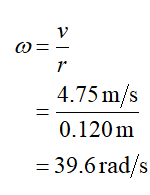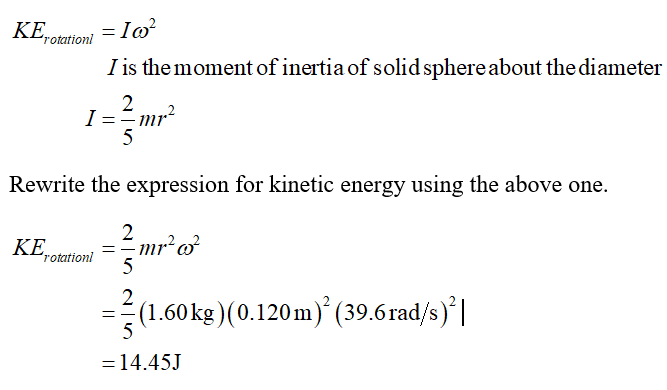# A uniform solid sphere with a radius of 0.120 m has a mass of 1.60 kg. The sphere is rolling along a horizontal surface, without slipping, at a constant speed of 4.75 m/s.a.  What is the rotational speed of the sphere about its center of mass?b.  What is the kinetic energy of the sphere due to its rotational motion about its center of mass?c.  What is the total kinetic energy of the sphere as it rolls without slipping?

Question
1 views

A uniform solid sphere with a radius of 0.120 m has a mass of 1.60 kg. The sphere is rolling along a horizontal surface, without slipping, at a constant speed of 4.75 m/s.

a.  What is the rotational speed of the sphere about its center of mass?

b.  What is the kinetic energy of the sphere due to its rotational motion about its center of mass?

c.  What is the total kinetic energy of the sphere as it rolls without slipping?

check_circle

a) Equation to find the rotational speed.a) Equation to find the rotational kinetic energy of solid sphere....

### Want to see the full answer?

See Solution

#### Want to see this answer and more?

Solutions are written by subject experts who are available 24/7. Questions are typically answered within 1 hour.*

See Solution
*Response times may vary by subject and question.
Tagged in